# Arrays and Iteration Arrays declaration and use single

• Slides: 34
Download presentation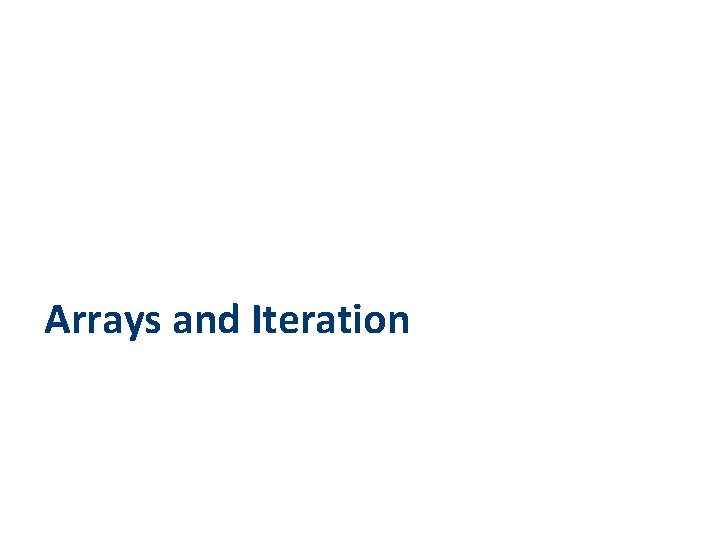Arrays and Iteration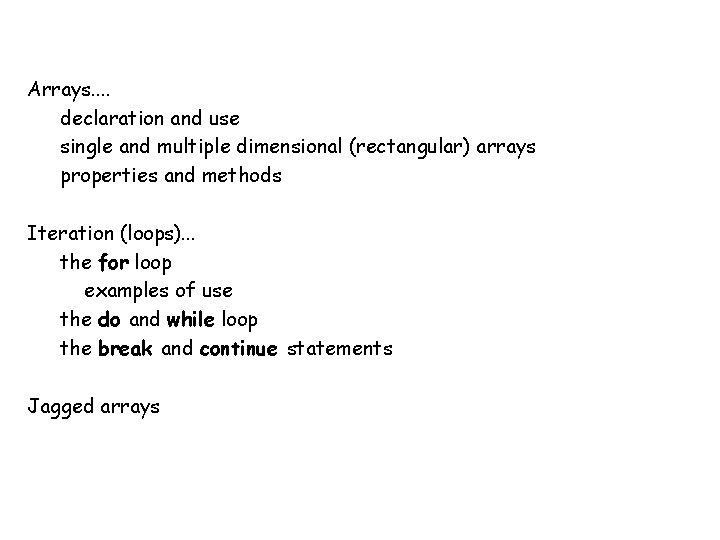Arrays. . declaration and use single and multiple dimensional (rectangular) arrays properties and methods Iteration (loops). . . the for loop examples of use the do and while loop the break and continue statements Jagged arrays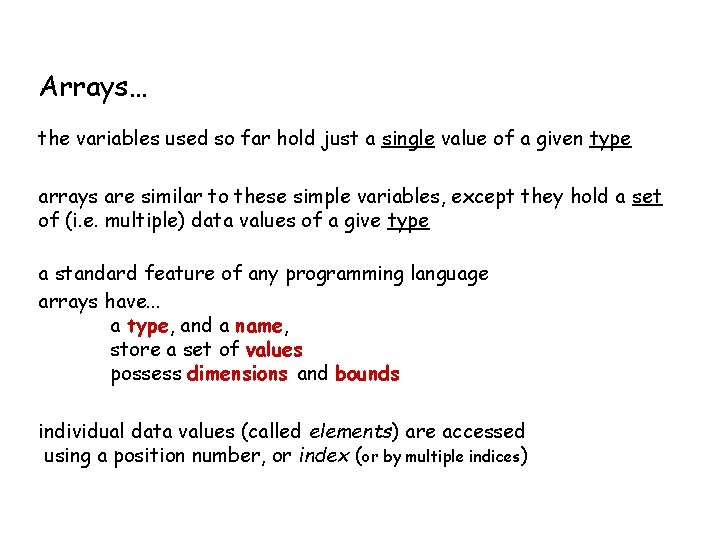Arrays… the variables used so far hold just a single value of a given type arrays are similar to these simple variables, except they hold a set of (i. e. multiple) data values of a give type a standard feature of any programming language arrays have. . . a type, and a name, store a set of values possess dimensions and bounds individual data values (called elements) are accessed using a position number, or index (or by multiple indices)simple variables versus arrays. . .string persons. Name; persons. Name = “jane”; using an array is only slightly more complex you must. . . specify its type give it a name determine its size and dimensions instantiate it ne “ja specify its type give it a name assign a value to it ” remember, to use a variable you must. . . pe rso a ns. N me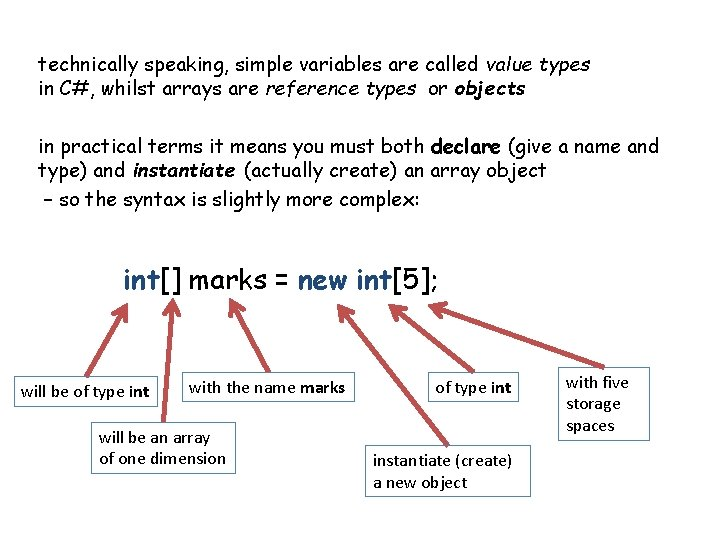technically speaking, simple variables are called value types in C#, whilst arrays are reference types or objects in practical terms it means you must both declare (give a name and type) and instantiate (actually create) an array object – so the syntax is slightly more complex: int[] marks = new int; will be of type int with the name marks will be an array of one dimension of type int instantiate (create) a new object with five storage spaces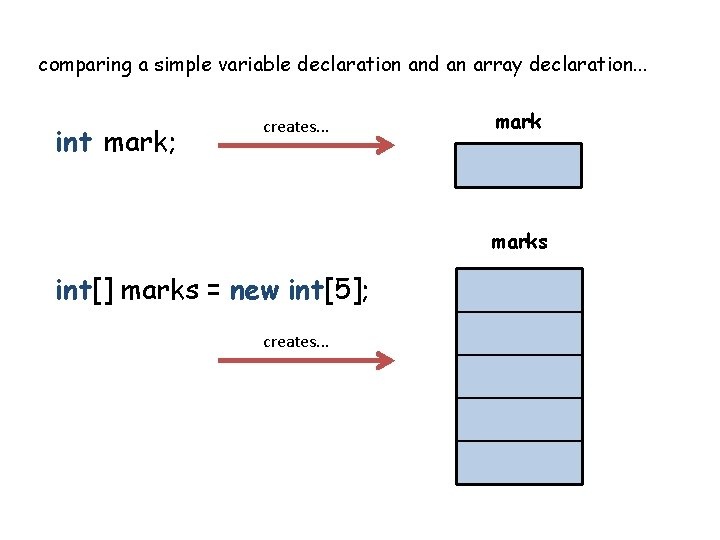comparing a simple variable declaration and an array declaration. . . int mark; creates. . . marks int[] marks = new int; creates. . .once an array has been declared and instantiated, how do you access the individual storage locations (called its elements). . . ? the elements are accessed using an index number mark = 25; leads to. . . mark 25 marks mark = 25; leads to. . . notice how the index numbers start from zero 0 0 0 1 0 2 25 3 0 4arrays are initialised with a default value (e. g. 0 for an int array), but you can initialise them with your own values too. . . int[] marks = new int {8, 7, 3, 9, 14}; marks leads to. . . 8 0 7 1 3 2 9 3 14 4why use arrays? arrays are great when you need to store and manipulate a set of data that are logically related – consider the example of storing a mark for each student that is enrolled on a module. we could use. . . int student 1 Mark; int student 2 Mark; … int student 85 Mark; so yes you could use a separate variable for each student, but it is clumsy and to do something like compute the average is intractable… ave_mark = (student 1 Mark + student 2 Mark + student 3 Mark + etc, + student 85 Mark) / 85 F;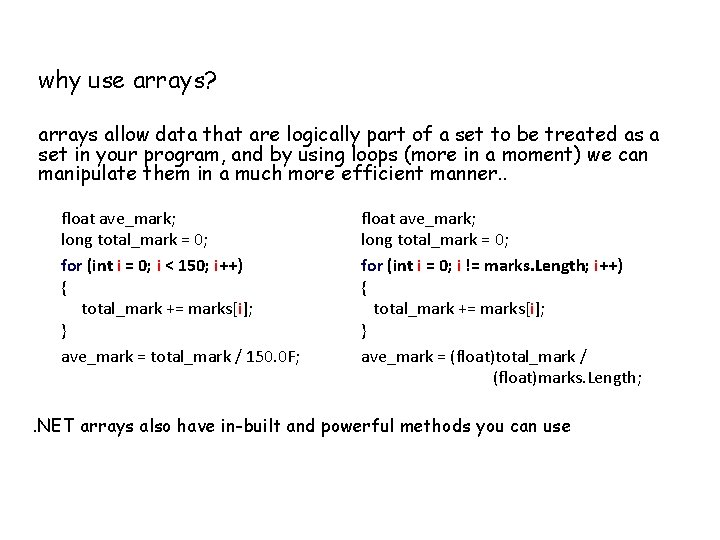why use arrays? arrays allow data that are logically part of a set to be treated as a set in your program, and by using loops (more in a moment) we can manipulate them in a much more efficient manner. . float ave_mark; long total_mark = 0; for (int i = 0; i < 150; i++) { total_mark += marks[i]; } ave_mark = total_mark / 150. 0 F; float ave_mark; long total_mark = 0; for (int i = 0; i != marks. Length; i++) { total_mark += marks[i]; } ave_mark = (float)total_mark / (float)marks. Length; . NET arrays also have in-built and powerful methods you can usenotes: scope rules are the same as for variables arrays are always zero-indexed in C# so if you declare an array of size n, the last element is item (n-1) all elements are of the same data type index values are enclosed by [square brackets] arrays are initialised with default values – e. g. O for type intmulti-dimensional (rectangular) arrays provide further structure to stored data – these arrays with more than one dimension. . . names leads to. . . string[] names = new string; int[, ] marks = new int[5, 2]; leads to. . . marks 0 0 0 1 0 0 2 0 0 3 0 0 4 0 1 Zebedee 0 Andrew 1 Fred 2 James 3 Tony 4an example of a 1 D (single) and a 2 D (multiple) storage array. . .array properties arrays have a number of useful properties and methods related to their size and dimensions… int[, ] marks = new int[5, 3]; marks. Rank gives. . . marks. Length gives. . . marks. Get. Length(0) marks. Get. Length(1) gives. . . marks. Get. Upper. Bound(0) marks. Get. Upper. Bound(1) gives. . . 2 15 5 3 4 2 marks 0 0 0 0 1 0 0 0 2 0 0 0 3 0 0 0 4 0 2 1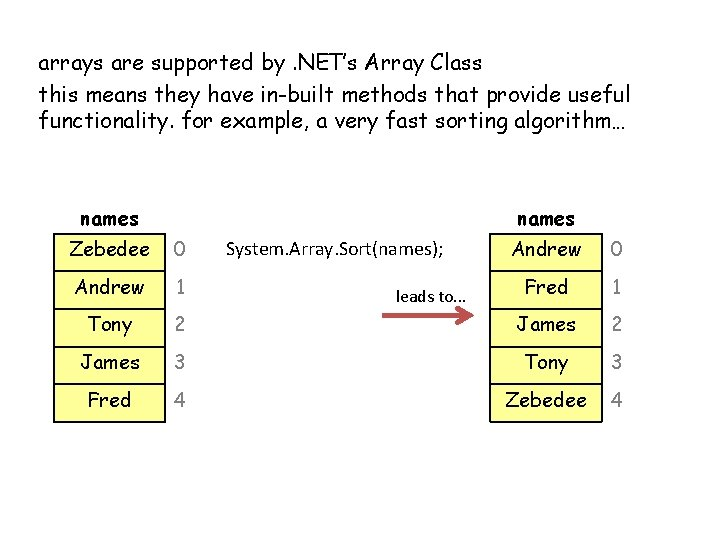arrays are supported by. NET’s Array Class this means they have in-built methods that provide useful functionality. for example, a very fast sorting algorithm… names Zebedee 0 Andrew 1 Fred 1 Tony 2 James 3 Tony 3 Fred 4 Zebedee 4 System. Array. Sort(names); leads to. . .you can even sort the elements of one array according to the values contained in another: both must be 1 -D and be of the same size System. Array. Sort(marks, names) names marks Zebedee 40 Andrew 90 Fred 33 James 62 Tony 55 System. Array. Sort(marks, names); leads to. . . names marks Fred 33 Zebedee 40 Tony 55 James 62 Andrew 90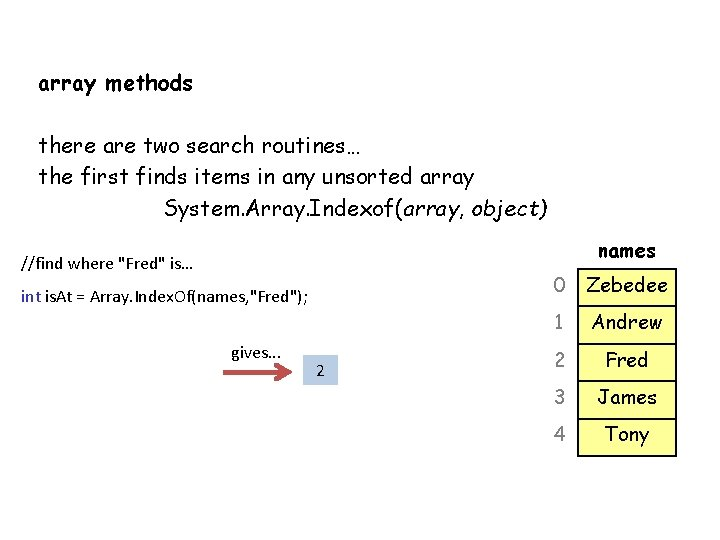array methods there are two search routines… the first finds items in any unsorted array System. Array. Indexof(array, object) names //find where "Fred" is… int is. At = Array. Index. Of(names, "Fred"); gives. . . 2 0 Zebedee 1 Andrew 2 Fred 3 James 4 Tonyarray methods the second needs a sorted array but is VERY fast System. Array. Binary. Search(array, object) names //find where "Fred" is… int is. At = Array. Binary. Seach(names, "Fred"); gives. . . 1 0 Andrew 1 Fred 2 James 3 Tony 4 Zebedee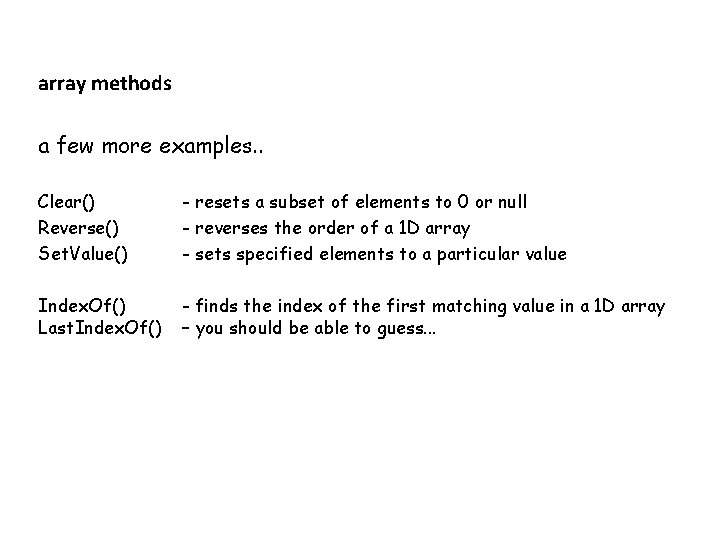array methods a few more examples. . Clear() Reverse() Set. Value() - resets a subset of elements to 0 or null - reverses the order of a 1 D array - sets specified elements to a particular value Index. Of() Last. Index. Of() - finds the index of the first matching value in a 1 D array – you should be able to guess. . .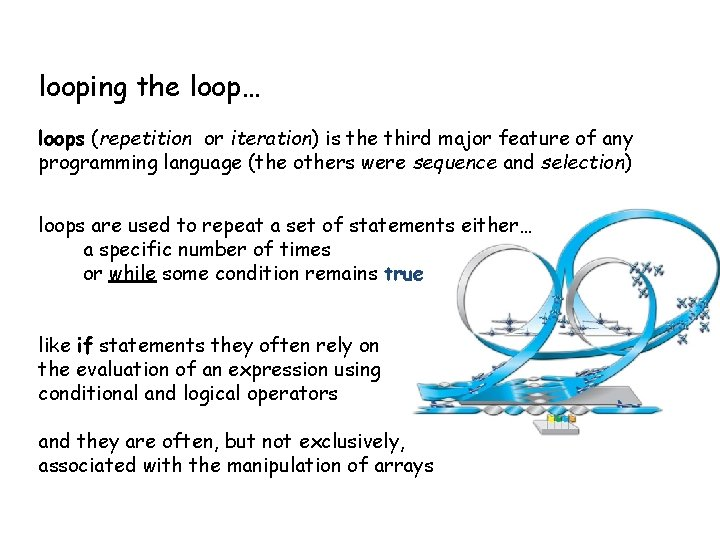looping the loop… loops (repetition or iteration) is the third major feature of any programming language (the others were sequence and selection) loops are used to repeat a set of statements either… a specific number of times or while some condition remains true like if statements they often rely on the evaluation of an expression using conditional and logical operators and they are often, but not exclusively, associated with the manipulation of arraysto repeat a set of statements a specific number of times. . . a for loop executes a statement block a specific number of times for (control ) { } general syntax fundamentally it is quite simple: the code to be repeated sits inside {brackets} and the tricky bit inside ( ) controls how many times the loop is repeated. . .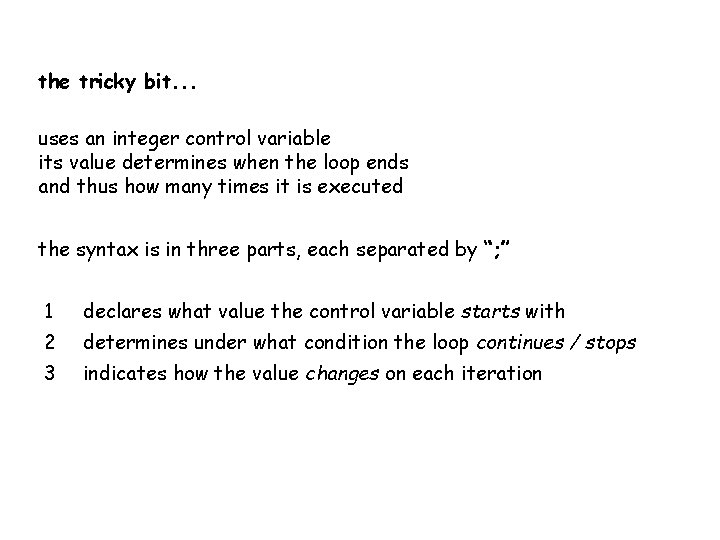the tricky bit. . . uses an integer control variable its value determines when the loop ends and thus how many times it is executed the syntax is in three parts, each separated by “; ” 1 declares what value the control variable starts with 2 determines under what condition the loop continues / stops 3 indicates how the value changes on each iterationexample. . . for (int i = 0; i < 10; i++) { //code to be repeated here } keyword for declares an int variable named i sets initial value of this control variable to zero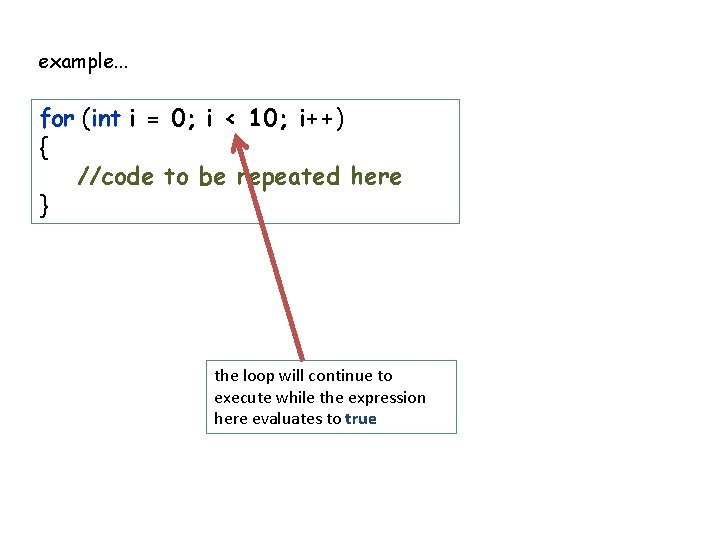example. . . for (int i = 0; i < 10; i++) { //code to be repeated here } the loop will continue to execute while the expression here evaluates to true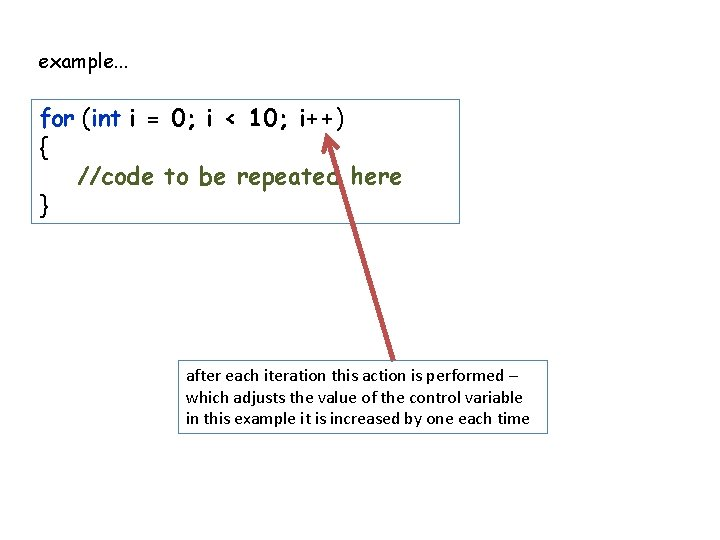example. . . for (int i = 0; i < 10; i++) { //code to be repeated here } after each iteration this action is performed – which adjusts the value of the control variable in this example it is increased by one each time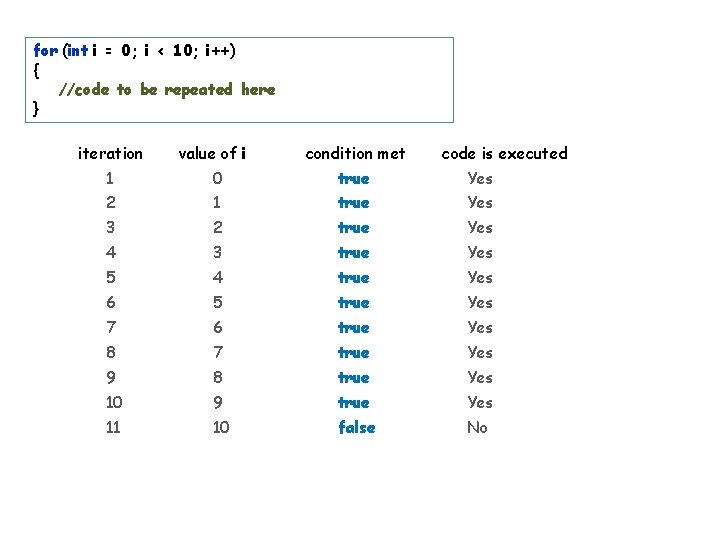for (int i = 0; i < 10; i++) { //code to be repeated here } iteration value of i condition met code is executed 1 0 true Yes 2 1 true Yes 3 2 true Yes 4 3 true Yes 5 4 true Yes 6 5 true Yes 7 6 true Yes 8 7 true Yes 9 8 true Yes 10 9 true Yes 11 10 false No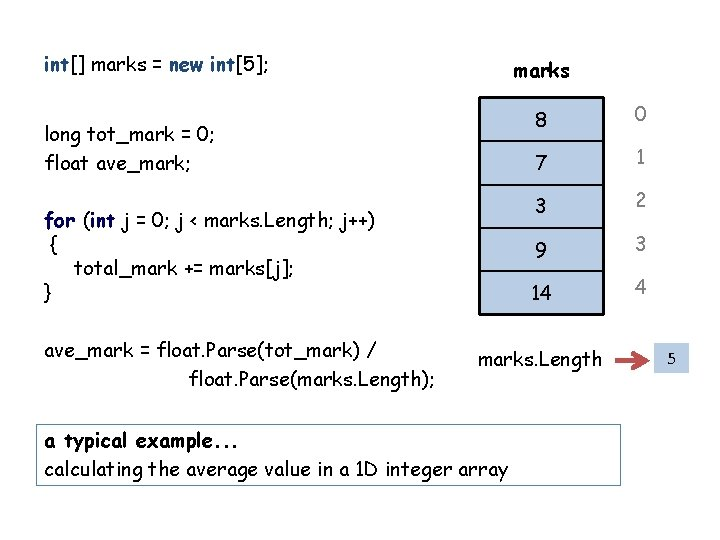int[] marks = new int; marks long tot_mark = 0; float ave_mark; for (int j = 0; j < marks. Length; j++) { total_mark += marks[j]; } ave_mark = float. Parse(tot_mark) / float. Parse(marks. Length); 8 0 7 1 3 2 9 3 14 4 marks. Length a typical example. . . calculating the average value in a 1 D integer array 5marks int max_mark = marks; int index_position = 0; 8 0 7 1 for (int j = 1; j<= marks. Get. Upperbound(0); j++) 3 { if (marks[j] > max_mark) 9 { 14 max_mark = marks[j]; index_position = j; } marks. Length } another example. . . finding the largest value, and its index, in a 1 D integer array 2 3 4 5notes: the end condition can be anything that eventually evaluates to false (if it doesn’t then the loop will never end) although ++ is most the common, the control update expression can be anything (e. g. i--, i +=2, i /=3, etc) although the control variables are often called i, j, k, etc. they need not be (but this is the one place where such names are acceptable) the control variable exists only while the loop is executing, so it cannot be used outside of the loopto repeat a set of statements while a specific condition is true. . . for this we use a do loop it has the same basic principle, executing a code block repeatedly, but this time if repeats as long as some condition is met do { //repeat code here } while ( true ) ; general syntax note the syntax carefully – particularly where “; ” appears the test occurs at the end of the code block, which means the code block is always executed at least onceto repeat a set of statements while a specific condition is true. . . we can also use the while loop very similar to a do loop, its just a matter of when the test is done while ( true ) { //repeat code here } general syntax note the syntax carefully – particularly that no “; ” appears the test occurs at the start of the code block, which means the code block may not be executed at all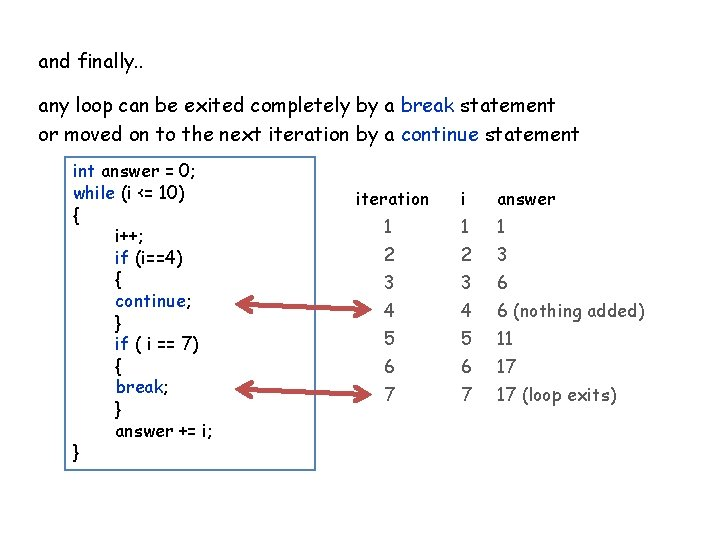and finally. . any loop can be exited completely by a break statement or moved on to the next iteration by a continue statement int answer = 0; while (i <= 10) { i++; if (i==4) { continue; } if ( i == 7) { break; } answer += i; } iteration i answer 1 1 1 2 2 3 3 3 6 4 4 6 (nothing added) 5 5 11 6 6 17 7 7 17 (loop exits)marks jagged arrays 23 55 40 multidimensional arrays are rectangular. . . int [, ] marks = new int [5, 3]; 72 80 90 8 12 33 19 60 62 47 44 55 jagged arrays are an array whose rows are also an array int[][] jmarks = new int[]; . . . read up for more details! jmarks 23 55 72 80 90 8 19 60 62 47 44